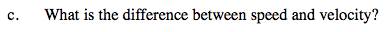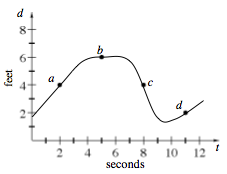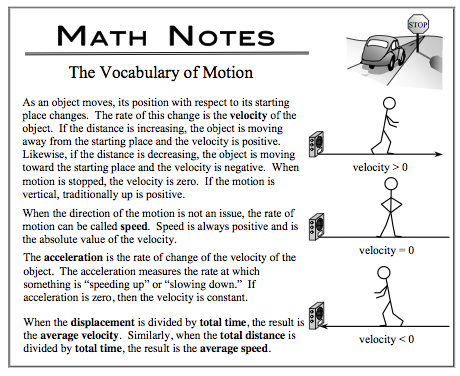### Home > CALC > Chapter 2 > Lesson 2.2.1 > Problem2-56

2-56.
1. Zuhaib is anxiously waiting for the results of his calculus test and is pacing back and forth as shown in the graph below. Homework Help ✎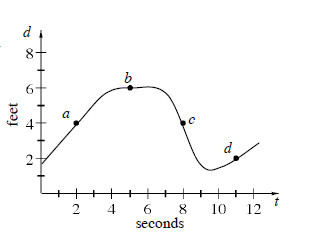1. At which point (a, b, c, or d) was Zuhaib's speed the greatest? Approximate the rate.

2. At which point was Zuhaib's velocity the greatest? Approximate the rate.

3. What is the difference between speed and velocity?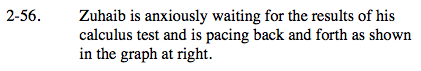This is a distance/position graph. Notice how Zuhaib is moving forward and backwards as she paces.

Speed is the absolute value of velocity. And velocity is the slope of a distance graph.

At which point is the absolute value of the slope the steepest?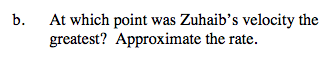Velocity is the slope on a distance/position graph.